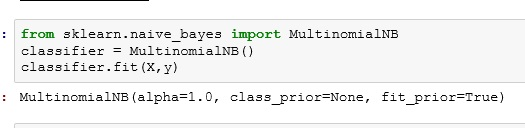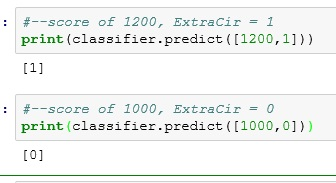# Python: Naive Bayes’

Naive Bayes’ is a supervised machine learning classification algorithm based off of Bayes’ Theorem. If you don’t remember Bayes’ Theorem, here it is:Seriously though, if you need a refresher, I have a lesson on it here: Bayes’ Theorem

The naive part comes from the idea that the probability of each column is computed alone. They are “naive” to what the other columns contain.

## Import the Data

```import pandas as pdLet’s look at the data. We have 3 columns – Score, ExtraCir, Accepted. These represent:

• Score – Student Test Score
• ExtraCir – Was Student in an Extra Circular Activity
• Accepted – Was the Student Accepted

Now the Accepted column is our result column – or the column we are trying to predict. Having a result in your data set makes this a supervised machine learning algorithm.

## Split the Data

Next split the data into input(score and extracir) and results (accepted).

```y = df.pop('Accepted')
X = df## Fit Naive Bayes

Lucky for us, scikitlearn has a bit in Naive Bayes algorithm – (MultinomialNB)

Import MultinomialNB and fit our split columns to it (X,y)

```from sklearn.naive_bayes import MultinomialNB
classifier = MultinomialNB()
classifier.fit(X,y)```## Run the some predictions

Let’s run the predictions below. The results show 1 (Accepted) 0 (Not Accepted)

```#--score of 1200, ExtraCir = 1
print(classifier.predict([1200,1]))

#--score of 1000, ExtraCir = 0
print(classifier.predict([1000,0]))```## The Code

```import pandas as pd

y = df.pop('Accepted')
X = df

from sklearn.naive_bayes import MultinomialNB
classifier = MultinomialNB()
classifier.fit(X,y)

#--score of 1200, ExtraCir = 1
print(classifier.predict([1200,1]))

#--score of 1000, ExtraCir = 0
print(classifier.predict([1000,0]))```

## One thought on “Python: Naive Bayes’”

1.shruthi

Hi when i run print(classifier.predict([1200,1])) i m getting the following error:

C:\Users\shruthibattula\AppData\Local\Continuum\anaconda2\lib\site-packages\sklearn\naive_bayes.py in predict(self, X)
64 Predicted target values for X
65 “””
—> 66 jll = self._joint_log_likelihood(X)
67 return self.classes_[np.argmax(jll, axis=1)]
68